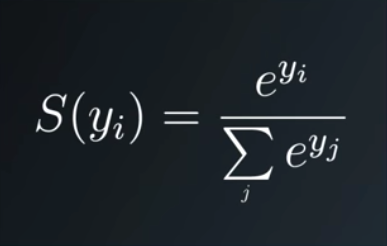top of page
Search
• Andrew Jones

# A simple explanation of how the Softmax function works

If you're versed in Neural Networks, you probably know the Softmax function as the function that takes your outputs and forces them all to add up to 1. It's extremely useful, and very commonly used in classification tasks.But how does it work it's magic exactly? It's quite simple when you break it down into it's parts.

Let's assume you have 5 classification scores being output from your Neural Network, one for each of the nodes in your final layer (I'll just make them up):

node_scores = [2.0, 0.1, 0.5, 1.0, 1.5]

What do we need to do to transform them, so they add up to 1 based on the Softmax function?

If we break the equation into two parts, we can follow it through.

The top layer of the equation takes Euler's number 'e' to the the power of each of our node score, i.e. e**2.0, e**0.1 and so on.

To do this in python we'll need to import numpy, and we can then do a list comprehension to run through the above calculation for each of our node scores.

import numpy as np eulerised_node_scores = [np.exp(i) for i in node_scores] print(eulerised_node_scores) > [7.389, 1.105, 1.648, 2.718, 4.481]

We then want to calculate the sum of our eulerised node scores:

sum_of_eulerised_node_scores = sum(eulerised_node_scores) print(sum_of_eulerised_node_scores) > 17.342919186503536

And finally, we divide each of our eulerised_node_scores by their sum and voila, we have our softmax scores:

softmax_scores = [j/sum_of_eulerised_node_scores for j in eulerised_node_scores] print(softmax_scores) > [0.426, 0.063, 0.095, 0.156, 0.258]

Let's check they add up to 1...

print(sum(softmax_scores)) > 1.0

I hope that was interesting, please do share to anyone else you think might be interested